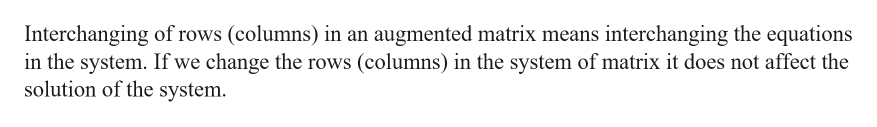# Explain why interchanging two rows of an augmented matrix results in an augmented matrix that represents an equivalent system of equations.

Question
14 views

Explain why interchanging two rows of an augmented matrix results in an augmented matrix that represents an equivalent system of equations.

check_circle

Step 1

Given:

Step 2

Explanation:help_outlineImage TranscriptioncloseInterchanging of rows (columns) in an augmented matrix means interchanging the equations in the system. If we change the rows (columns) in the system of matrix it does not affect the solution of the system. fullscreen
Step 3

Example:

...

### Want to see the full answer?

See Solution

#### Want to see this answer and more?

Solutions are written by subject experts who are available 24/7. Questions are typically answered within 1 hour.*

See Solution
*Response times may vary by subject and question.
Tagged in

### Matrices